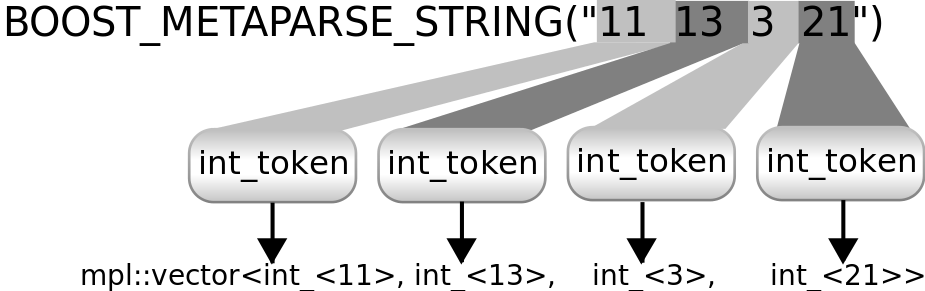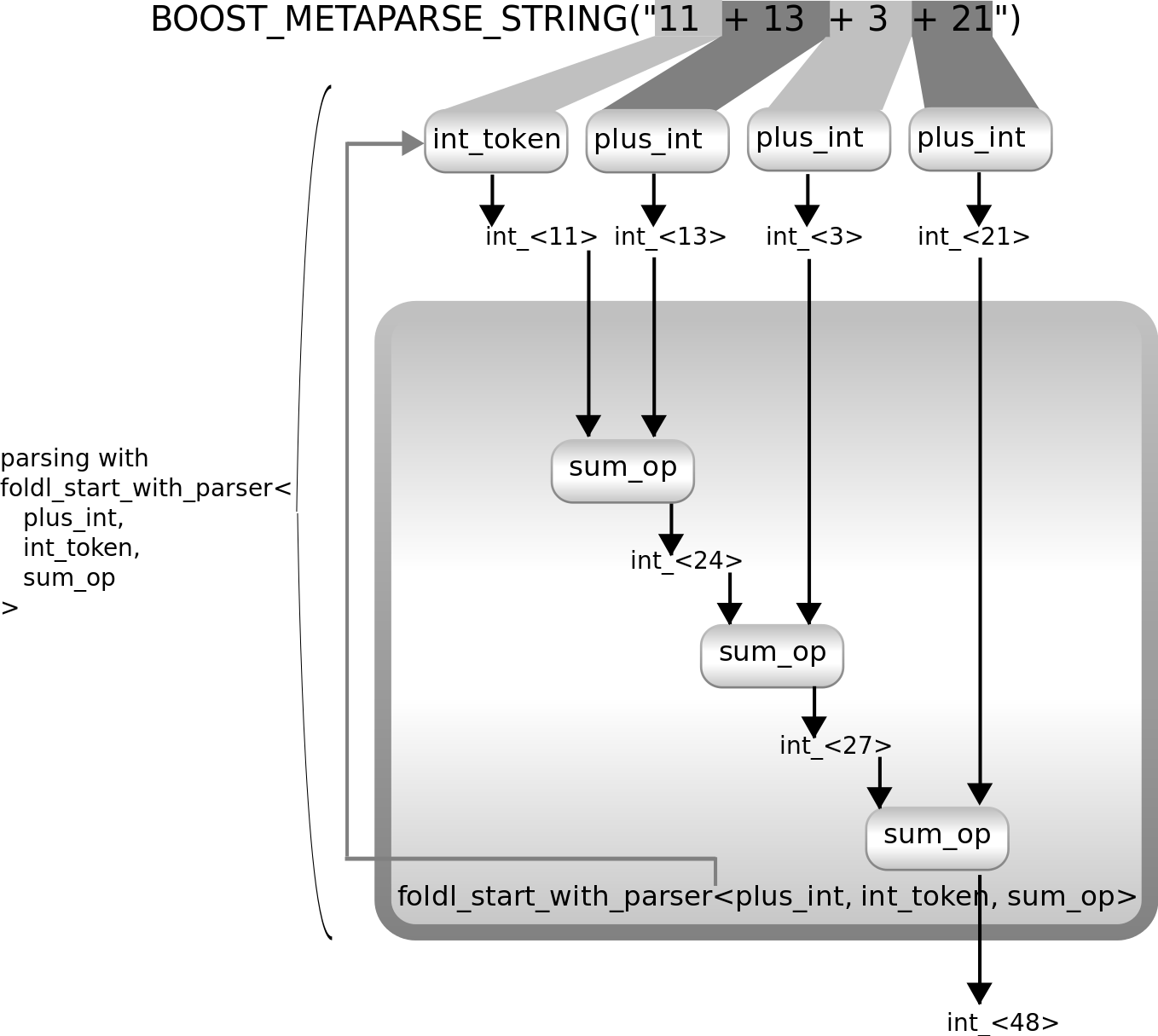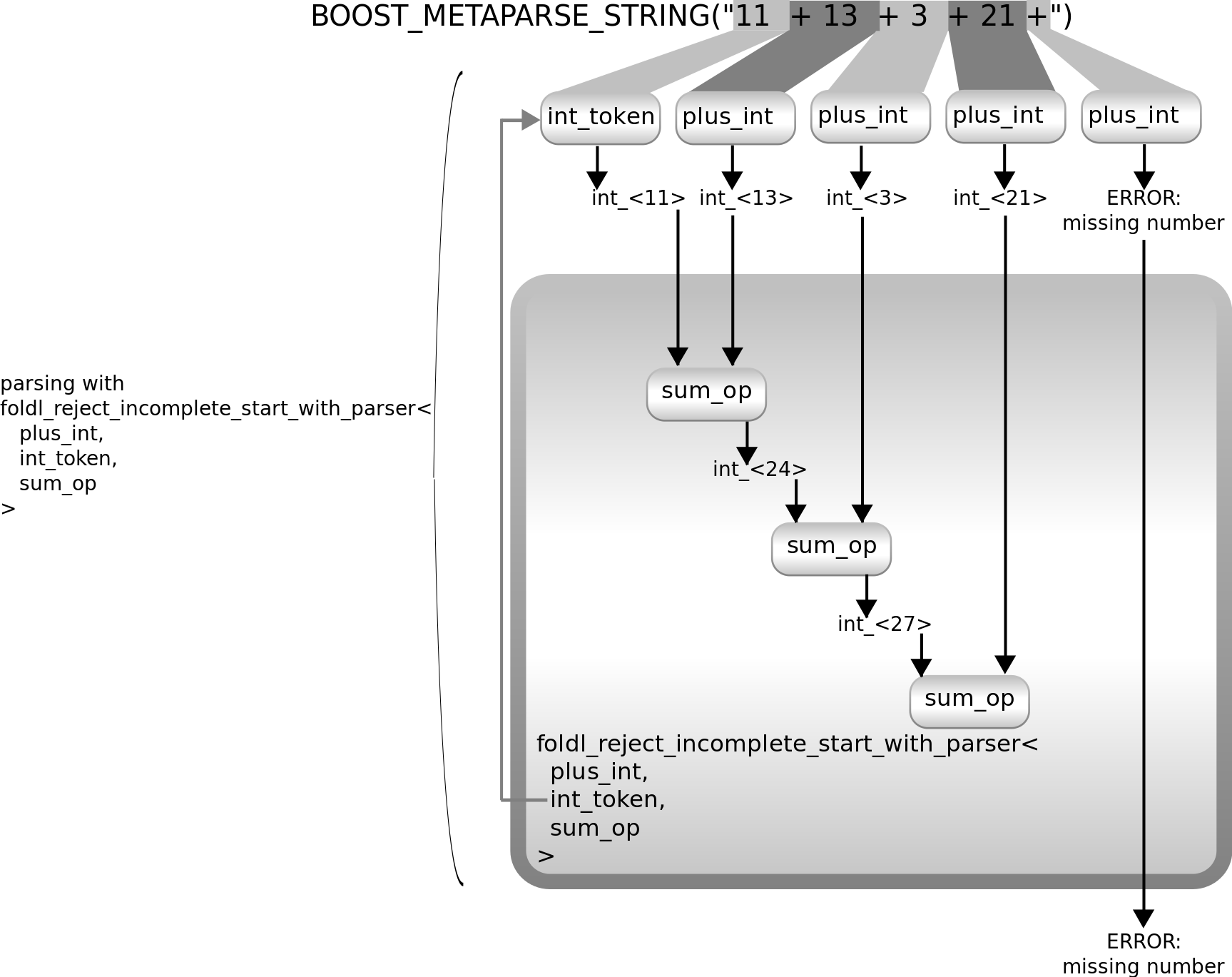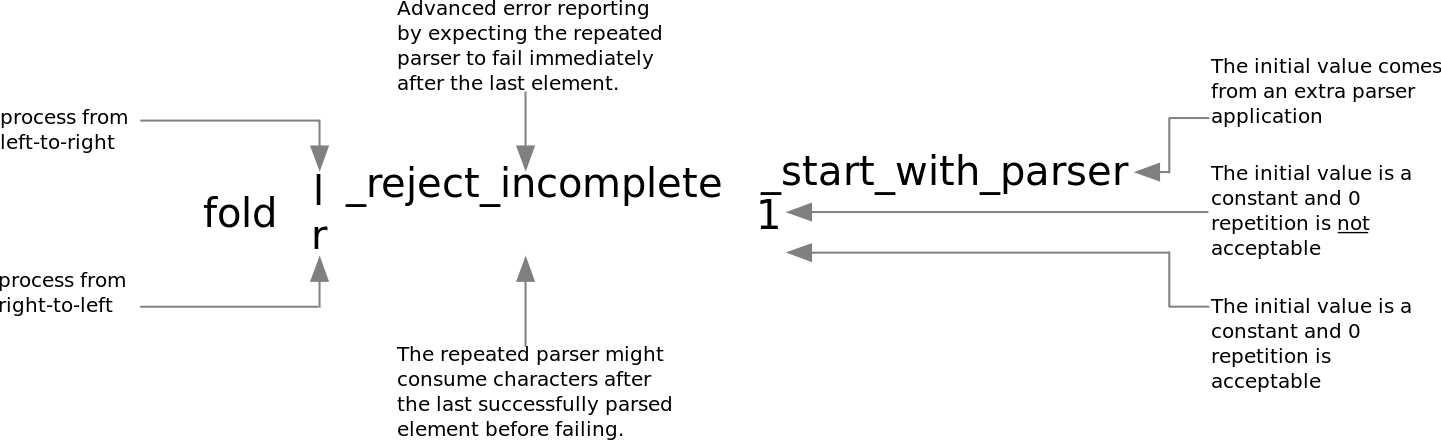#Boost C++ Libraries

...one of the most highly regarded and expertly designed C++ library projects in the world.

## User manual

What is a parser
Parsing based on `constexpr`
What types of grammars can be used?

### What is a parser

See the parser section of the reference for the explanation of what a parser is.

#### The input of the parsers

Parsers take a `string` as input, which represents a string for template metaprograms. For example the string `"Hello World!"` can be defined the following way:

```string<'H','e','l','l','o',' ','W','o','r','l','d','!'>
```

This syntax makes the input of the parsers difficult to read. Metaparse works with compilers using C++98, but the input of the parsers has to be defined the way it is described above.

Based on `constexpr`, a feature provided by C++11, Metaparse provides a macro, `BOOST_METAPARSE_STRING` for defining strings:

```BOOST_METAPARSE_STRING("Hello World!")
```

This defines a `string` as well, however, it is easier to read. The maximum length of the string that can be defined this way is limited, however, this limit is configurable. It is specified by the `BOOST_METAPARSE_LIMIT_STRING_SIZE` macro.

#### Source positions

A source position is described using a compile-time data structure. The following functions can be used to query it:

The beginning of the input is `start` which requires `<boost/metaparse/start.hpp>` to be included.

#### Error handling

An error is described using a compile-time data structure. It contains information about the source position where the error was detected and some description about the error. `debug_parsing_error` can be used to display the error message. Metaparse provides the `BOOST_METAPARSE_DEFINE_ERROR` macro for defining simple parsing error messages.

#### Combining parsers

Complex parsers can be built by combining simple parsers. The parser library contains a number of parser combinators that build new parsers from already existing ones.

For example `accept_when``<Parser, Predicate, RejectErrorMsg>` is a parser. It uses `Parser` to parse the input. When `Parser` rejects the input, the combinator returns the error `Parser` failed with. When `Parser` is successful, the combinator validates the result using `Predicate`. If the predicate returns true, the combinator accepts the input, otherwise it generates an error with the message `RejectErrorMsg`.

Having `accept_when`, `one_char` can be used to build parsers that accept only digit characters, only whitespaces, etc. For example `digit` accepts only digit characters:

```typedef
boost::metaparse::accept_when<
boost::metaparse::one_char,
boost::metaparse::util::is_digit,
boost::metaparse::errors::digit_expected
>
digit;
```

#### Sequence

The result of a successful parsing is some value and the remaining string that was not parsed. The remaining string can be processed by another parser. The parser library provides a parser combinator, `sequence`, that takes a number of parsers as arguments and builds a new parser from them that:

• Parses the input using the first parser
• If parsing succeeds, it parses the remaining string with the second parser
• It continues applying the parsers in order as long as they succeed
• If all of them succeed, it returns the list of results
• If any of the parsers fails, the combinator fails as well and returns the error the first failing parser returned with

#### Repetition

It is a common thing to parse a list of things of unknown length. As an example let's start with something simple: the text is a list of numbers. For example:

```11 13 3 21
```

We want the result of parsing to be the sum of these values. Metaparse provides the `int_` parser we can use to parse one of these numbers. Metaparse provides the `token` combinator to consume the whitespaces after the number. So the following parser parses one number and the whitespaces after it:

```using int_token = token<int_>;
```

The result of parsing is a boxed integer value: the value of the parsed number. For example parsing `BOOST_METAPARSE_STRING``("13 ")` gives `boost::mpl::int_<13>` as the result.

Our example input is a list of numbers. Each number can be parsed by `int_token`:This diagram shows how the repeated application of `int_token` can parse the example input. Metaparse provides the `repeated` parser to easily implement this. The result of parsing is a typelist: the list of the individual numbers.This diagram shows how `repeated``<int_token>` works. It uses the `int_token` parser repeatedly and builds a `boost::mpl::vector` from the results it provides.

But we need the sum of these, so we need to summarise the result. We can do this by wrapping our parser, `repeated``<int_token>` with `transform`. That gives us the opportunity to specify a function transforming this typelist to some other value - the sum of the elements in our case. Initially let's ignore how to summarise the elements in the vector. Let's assume that it can be implemented by a lambda expression and use `boost::mpl::lambda<...>::type` representing that lambda expression. Here is an example using `transform` and this lambda expression:

```using sum_parser =
transform<
repeated<int_token>,
boost::mpl::lambda<...>::type
>;
```

The `transform``<>` parser combinator wraps the `repeated``<int_token>` to build the parser we need. Here is a diagram showing how it works:As the diagram shows, the `transform``<``repeated````<int_token>, ...>``` parser parses the input using `repeated``<int_token>` and then does some processing on the result of parsing.

Let's implement the missing lambda expression that tells `transform` how to change the result coming from `repeated``<int_token>`. We can summarise the numbers in a typelist by using Boost.MPL's `fold` or `accumulate`. Here is an example doing that:

```using sum_op = mpl::lambda<mpl::plus<mpl::_1, mpl::_2>>::type;

using sum_parser =
transform<
repeated<int_token>,
mpl::lambda<
mpl::fold<mpl::_1, mpl::int_<0>, sum_op>
>::type
>;
```

Here is an extended version of the above diagram showing what happens here:This example parses the input, builds the list of numbers and then loops over it and summarises the values. It starts with the second argument of `fold`, `int_<0>` and adds every item of the list of numbers (which is the result of the parser `repeated``<int_token>`) one by one.

NoteNote that `transform` wraps another parser, `repeated``` here. It parses the input with that parser, gets the result of that parsing and changes that result. `transform` itself will be a parser returning that updated result.
##### Introducing foldl

It works, however, this is rather inefficient: it has a loop parsing the integers one by one, building a typelist and then it loops over this typelist to summarise the result. Using template metaprograms in your applications can have a serious impact on the compiler's memory usage and the speed of the compilation, therefore I recommend being careful with these things.

Metaparse offers more efficient ways of achieving the same result. You don't need two loops: you can merge them together and add every number to your summary right after parsing it. Metaparse offers the `foldl` for this.

With `foldl` you specify:

• the parser to parse the individual elements of the list (which is `int_token` in our example)
• the initial value used for folding (which is `int_<0>` in our example)
• the forward operation merging the sub-result we have so far and the value coming from the last application of the parser (this was `sum_op` in our example)

Our parser can be implemented this way:

```using better_sum_parser = foldl<int_token, mpl::int_<0>, sum_op>;
```

As you can see the implementation of the parser is more compact. Here is a diagram showing what happens when you use this parser to parse some input:As you can see, not only the implementation of the parser is more compact, but it achieves the same result by doing less as well. It parses the input by applying `int_token` repeatedly, just like the previous solution. But it produces the final result without building a typelist as an internal step. Here is how it works internally:It summarises the results of the repeated `int_token` application using `sum_op`. This implementation is more efficient. It accepts an empty string as a valid input: the sum of it is `0`. It may be good for you, in which case you are done. If you don't wan to accept it, you can use `foldl1` instead of `foldl`. This is the same, but it rejects empty input. (Metaparse offers `repeated1` as well if you choose the first approach and would like to reject empty string)

##### Introducing foldr
NoteNote that if you are reading this manual for the first time, you probably want to skip this section and proceed with Introducing foldl_start_with_parser

You might have noticed that Metaparse offers `foldr` as well. The difference between `foldl` and `foldr` is the direction in which the results are summarised. (`l` stands for from the Left and `r` stands for from the Right) Here is a diagram showing how `better_sum_parser` works if it is implemented using `foldr`:As you can see this is very similar to using `foldl`, but the results coming out of the individual applications of `int_token` are summarised in a right-to-left order. As `sum_op` is addition, it does not affect the end result, but in other cases it might.

NoteNote that the implementation of `foldl` is more efficient than `foldr`. Prefer `foldl` whenever possible.

As you might expect it, Metaparse offers `foldr1` as well, which folds from the right and rejects empty input.

##### Introducing foldl_start_with_parser

Let's change the grammar of our little language. Instead of a list of numbers, let's expect numbers separated by a `+` symbol. Our example input becomes the following:

```BOOST_METAPARSE_STRING("11 + 13 + 3 + 21")
```

Parsing it with `foldl` or `repeated` is difficult: there has to be a `+` symbol before every element except the first one. None of the already introduced repetition constructs offer a way of treating the first element in a different way.

If we forget about the first number for a moment, the rest of the input is `"+ 13 + 3 + 21"`. This can easily be parsed by `foldl` (or `repeated`):

```using plus_token = token<lit_c<'+'>>;
using plus_int = last_of<plus_token, int_token>;

using sum_parser2 = foldl<plus_int, int_<0>, sum_op>;
```

It uses `plus_int`, that is `last_of``<plus_token, int_token>` as the parser that is used repeatedly to get the numbers. It does the following:

• Uses `plus_token` to parse the `+` symbol and any whitespace that might follow it.
• Uses then `int_token` to parse the number
• Combines the above two with `last_of` to use both parsers in order and keep only the result of using the second one (the result of parsing the `+` symbol is thrown away - we don't care about it).

This way `last_of``<plus_token, int_token>` returns the value of the number as the result of parsing, just like our previous parser, `int_token` did. Because of this, it can be used as a drop-in replacement of `int_token` in the previous example and we get a parser for our updated language. Or at least for all number except the first one.

This `foldl` can not parse the first element, because it expects a `+` symbol before every number. You might think of making the `+` symbol optional in the above approach - don't do that. It makes the parser accept ```"11 + 13 3 21"``` as well as the `+` symbol is now optional everywhere.

What you could do is parsing the first element with `int_token`, the rest of the elements with the above `foldl`-based solution and add the result of the two. This is left as an exercise to the reader.

Metaparse offers `foldl_start_with_parser` to implement this. `foldl_start_with_parser` is the same as `foldl`. The difference is that instead of an initial value to combine the list elements with it takes an initial parser:

```using plus_token = token<lit_c<'+'>>;
using plus_int = last_of<plus_token, int_token>;

using sum_parser3 = foldl_start_with_parser<plus_int, int_token, sum_op>;
```

`foldl_start_with_parser` starts with applying that initial parser and uses the result it returns as the initial value for folding. It does the same as `foldl` after that. The following diagram shows how it can be used to parse a list of numbers separated by `+` symbols:As the diagram shows, it start parsing the list of numbers with `int_token`, uses its value as the starting value for folding (earlier approaches were using the value `int_<0>` as this starting value). Then it parses all elements of the list by using `plus_int` multiple times.

##### Introducing foldr_start_with_parser
NoteNote that if you are reading this manual for the first time, you probably want to skip this section and try creating some parsers using `foldl_start_with_parser` instead.

`foldl_start_with_parser` has its from the right pair, `foldr_start_with_parser`. It uses the same elements as `foldl_start_with_parser` but in a different order. Here is a parser for our example language implemented with `foldr_start_with_parser`:

```using plus_token = token<lit_c<'+'>>;
using int_plus = first_of<int_token, plus_token>;

using sum_parser4 = foldr_start_with_parser<int_plus, int_token, sum_op>;
```

Note that it uses `int_plus` instead of `plus_int`. This is because the parser the initial value for folding comes from is used after `int_plus` has parsed the input as many times as it could. It might sound strange for the first time, but the following diagram should help you understand how it works:As you can see, it starts with the parser that is applied repeatedly on the input, thus instead of parsing ```plus_token int_token``` repeatedly, we need to parse `int_token plus_token` repeatedly. The last number is not followed by `+`, thus `int_plus` fails to parse it and it stops the iteration. `foldr_start_with_parser` then uses the other parser, `int_token` to parse the input. It succeeds and the result it returns is used as the starting value for folding from the right.

NoteNote that as the above description also suggests, the implementation of `foldl_start_with_parser` is more efficient than `foldr_start_with_parser`. Prefer `foldl_start_with_parser` whenever possible.
##### Introducing foldl_reject_incomplete_start_with_parser

Using a parser built with `foldl_start_with_parser` we can parse the input when the input is correct. However, it is not always the case. Consider the following input for example:

```BOOST_METAPARSE_STRING("11 + 13 + 3 + 21 +")
```

This is an invalid expression. However, if we parse it using the `foldl_start_with_parser`-based parser presented earlier (`sum_parser3`), it accepts the input and the result is `48`. This is because `foldl_start_with_parser` parses the input as long as it can. It parses the first`int_token` (`11`) and then it starts parsing the `plus_int` elements (`+ 13`, `+ 3`, `+ 21`). After parsing all of these, it tries to parse the remaining `" +"` input using `plus_int` which fails and therefore `foldl_start_with_parser` stops after `+ 21`.

The problem is that the parser parses the longest sub-expression starting from the beginning, that represents a valid expression. The rest is ignored. The parser can be wrapped by `entire_input` to make sure to reject expressions with invalid extra characters at the end, however, that won't make the error message useful. (`entire_input` can only tell the author of the invalid expression that after ```+ 21``` is something wrong).

Metaparse provides `foldl_reject_incomplete_start_with_parser`, which does the same as `foldl_start_with_parser`, except that once no further repetitions are found, it checks where the repeated parser (in our example `plus_int`) fails. When it can make any progress (eg. it finds a `+` symbol), then `foldl_reject_incomplete_start_with_parser` assumes, that the expression's author intended to make the repetition longer, but made a mistake and propagates the error message coming from that last broken expression.The above diagram shows how `foldl_reject_incomplete_start_with_parser` parses the example invalid input and how it fails. This can be used for better error reporting from the parsers.

Other folding parsers also have their `f` version. (eg. `foldr_reject_incomplete`, `foldl_reject_incomplete1`, etc).

##### Finding the right folding parser combinator

As you might have noticed, there are a lot of different folding parser combinators. To help you find the right one, the following naming convention is used:NoteNote that there is no `foldr_reject_incomplete_start_with_parser`. The `p` version of the right-folding parsers applies the special parser, whose result is the initial value, after the repeated elements. Therefore, when the parser parsing one repeated element fails, `foldr_start_with_parser` would apply that special final parser instead of checking how the repeated element's parser failed.

#### What can be built from a compile-time string?

Parsers built using Metaparse are template metaprograms parsing text (or code) at compile-time. Here is a list of things that can be the "result" of parsing:

• A type. An example for this is a parser parsing a `printf` format string and returning the typelist (eg. `boost::mpl::vector`) of the expected arguments.
• A constant value. An example for this is the result of a calculator language. See the Getting Started section for further details.
• A runtime object. A static runtime object can be generated that might be used at runtime. An example for this is parsing regular expressions at compile-time and building `boost::xpressive::sregex` objects. See the `regex` example of Metaparse for an example.
• A C++ function, which might be called at runtime. A C++ function can be generated that can be called at runtime. It is good for generating native (and optimised) code from EDSLs. See the `compile_to_native_code` example of Metaparse as an example for this.
• A template metafunction class. The result of parsing might be a type, which is a template metafunction class. This is good for building an EDSL for template metaprogramming. See the `meta_hs` example of Metaparse as an example for this.

#### Grammars

Metaparse provides a way to define grammars in a syntax that resembles EBNF. The `grammar` template can be used to define a grammar. It can be used the following way:

```grammar<BOOST_METAPARSE_STRING("plus_exp")>
::import<BOOST_METAPARSE_STRING("int_token"), token<int_>>::type

::rule<BOOST_METAPARSE_STRING("ws ::= (' ' | '\n' | '\r' | '\t')*")>::type
::rule<BOOST_METAPARSE_STRING("plus_token ::= '+' ws"), front<_1>>::type
::rule<BOOST_METAPARSE_STRING("plus_exp ::= int_token (plus_token int_token)*"), plus_action>::type
```

The code above defines a parser from a grammar definition. The start symbol of the grammar is `plus_exp`. The lines beginning with `::rule` define rules. Rules optionally have a semantic action, which is a metafunction class that transforms the result of parsing after the rule has been applied. Existing parsers can be bound to names and be used in the rules by importing them. Lines beginning with `::import` bind existing parsers to names.

The result of a grammar definition is a parser which can be given to other parser combinators or be used directly. Given that grammars can import existing parsers and build new ones, they are parser combinators as well.

### Parsing based on `constexpr`

Metaparse is based on template metaprogramming, however, C++11 provides `constexpr`, which can be used for parsing at compile-time as well. While implementing parsers based on `constexpr` is easier for a C++ developer, since its syntax resembles the regular syntax of the language, the result of parsing has to be a `constexpr` value. Parsers based on template metaprogramming can build types as the result of parsing. These types may be boxed `constexpr` values but can be metafunction classes, classes with static functions which can be called at runtime, etc.

When a parser built with Metaparse needs a sub-parser for processing a part of the input text and generating a `constexpr` value as the result of parsing, one can implement the sub-parser based on `constexpr` functions. Metaparse can be integrated with them and lift their results into C++ template metaprogramming. An example demonstrating this feature can be found among the examples (`constexpr_parser`). This capability makes it possible to integrate Metaparse with parsing libraries based on `constexpr`.

### What types of grammars can be used?

It is possible to write parsers for context free grammars using Metaparse. However, this is not the most general category of grammars that can be used. As Metaparse is a highly extendable framework, it is not clear what should be considered to be the limit of Metaparse itself. For example Metaparse provides the `accept_when` parser combinator. It can be used to provide arbitrary predicates for enabled/disabling a specific rule. One can go as far as providing the Turing machine (as a metafunction) of the entire grammar as a predicate, so one can build parsers for unrestricted grammars that can be parsed using a Turing machine. Note that such a parser would not be considered to be a parser built with Metaparse, however, it is not clear how far a solution might go and still be considered using Metaparse.

Metaparse assumes that the parsers are deterministic, as they have only "one" result. It is of course possible to write parsers and combinators that return a set (or list or some other container) of results as that "one" result, but that can be considered building a new parser library. There is no clear boundary for Metaparse.

Metaparse supports building top-down parsers and left-recursion is not supported as it would lead to infinite recursion. Right-recursion is supported, however, in most cases the iterative parser combinators provide better alternatives.